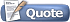Thread: Sampling .wav files View Single Post09-18-2014, 10:34 AM #13 Alkamist Human being with feelings   Join Date: Dec 2011 Posts: 495So I'm trying to make a function that puts the sample in a left and right buffer, but I'm having issues. I'm using vectors for my buffers because I'm not sure I can do it any other way, since the vector needs to change its size depending on how big the sound data is. in Sampler.h Code: #ifndef __SAMPLER__ #define __SAMPLER__ #include #include #include class Sampler { public: ifstream myfile; void LoadWaveFile(char *fname); void BufferSample(); void NextSample(double &leftOutput, double &rightOutput); Sampler(void); ~Sampler(void); private: char *mChunkID, *mChunkSize, *mFormat, *mSubChunkOneID, *mSubChunkOneSize, *mAudioFormat, *mNumChannels, *mSampleRate, *mByteRate, *mBlockAlign, *mBitsPerSample, *mSubChunkTwoID, *mSubChunkTwoSize, *mData; std::vector mOutputL(); std::vector mOutputR(); }; #endif /* defined(__SAMPLER__) */ in Sampler.cpp Code: void Sampler::BufferSample() { mOutputL.resize(*mSubChunkTwoSize/2, 0); mOutputR.resize(*mSubChunkTwoSize/2, 0); short int *dataPT; dataPT = (short int *)mData; for (int i = 0, int j = 0; i < *mSubChunkTwoSize, j < *mSubChunkTwoSize/2; i += 2, j++) { if (dataPT[i] >= 0) { mOutputL[j] = dataPT[i]/32767; } else { mOutputL[j] = dataPT[i]/32768; } if (dataPT[i+1] >= 0) { mOutputR[j] = dataPT[i+1]/32767; } else { mOutputR[j] = dataPT[i+1]/32768; } } } It's telling me for all of the cases that I'm trying to use the vectors that they need to have a class type. Any idea what I'm doing wrong?